# Types of Chemical Formulas Types of Chemical Formulas

• Slides: 14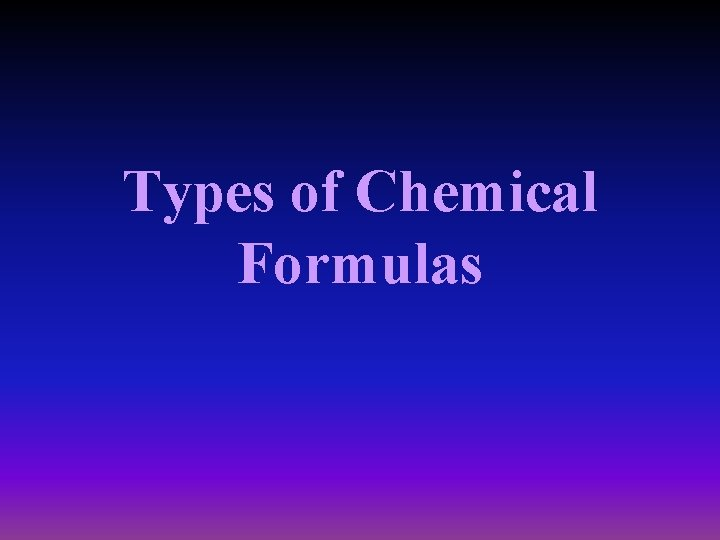Types of Chemical FormulasTypes of Chemical Formulas • Molecular – number of atoms present in the smallest particle of that compound – C 6 H 12 O 6 • Empirical – simplest whole number ratio of atoms in a compound – CH 2 O• To go from molecular C 6 H 12 O 6 to empirical CH 2 O as seen on the last side…. . • just reduce or simplify the coefficients as you would a fraction. • Keep in mind: the coefficients must be in the same ratio. • C 6 H 12 O 6 all coefficients are divisible by 2.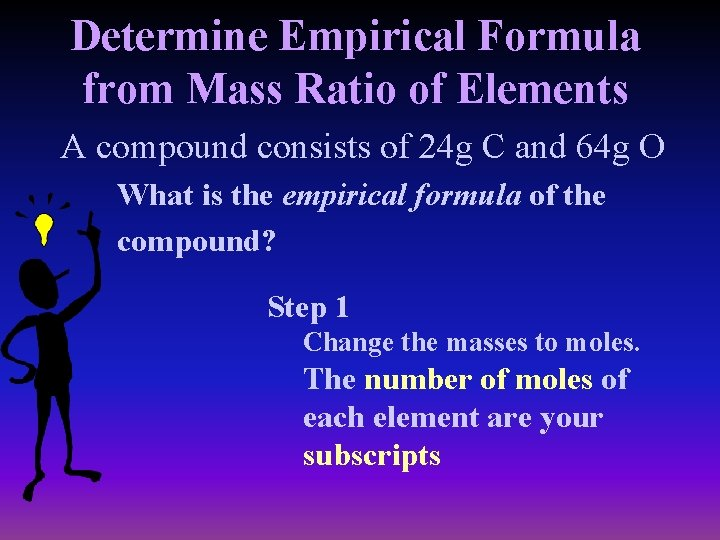Determine Empirical Formula from Mass Ratio of Elements A compound consists of 24 g C and 64 g O What is the empirical formula of the compound? Step 1 Change the masses to moles. The number of moles of each element are your subscripts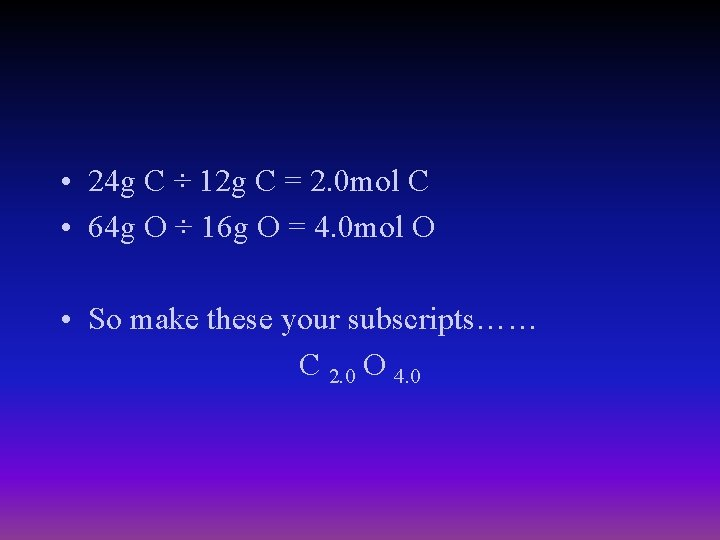• 24 g C ÷ 12 g C = 2. 0 mol C • 64 g O ÷ 16 g O = 4. 0 mol O • So make these your subscripts…… C 2. 0 O 4. 0• Step 2 – subscripts must be smallest whole numbers – C 2. 0 O 4. 0 reduces to CO 2Practice…what is the empirical formula? • A pure sample contains 14 g N and 8 g O N 2 O • A compound consists of 36. 0 g C and 12. 0 g H CH 4 • A substance consists of 52 g Cr and 24 g O Cr 2 O 3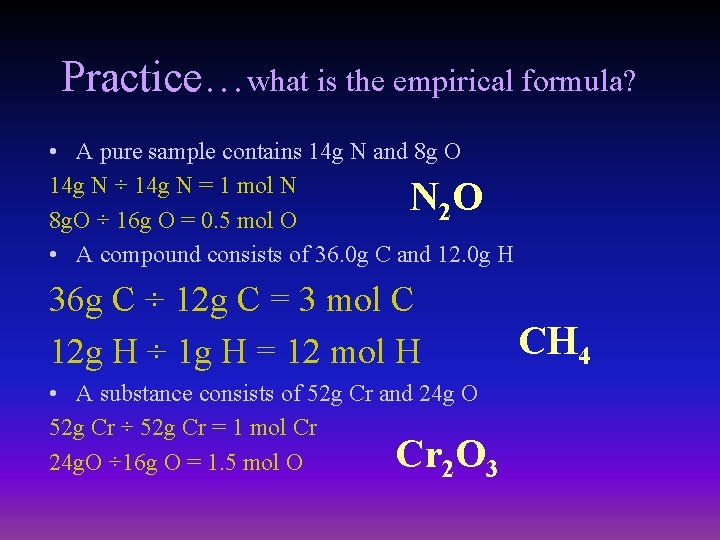Practice…what is the empirical formula? • A pure sample contains 14 g N and 8 g O 14 g N ÷ 14 g N = 1 mol N 2 8 g. O ÷ 16 g O = 0. 5 mol O • A compound consists of 36. 0 g C and 12. 0 g H NO 36 g C ÷ 12 g C = 3 mol C 12 g H ÷ 1 g H = 12 mol H • A substance consists of 52 g Cr and 24 g O 52 g Cr ÷ 52 g Cr = 1 mol Cr 24 g. O ÷ 16 g O = 1. 5 mol O 2 3 Cr O CH 4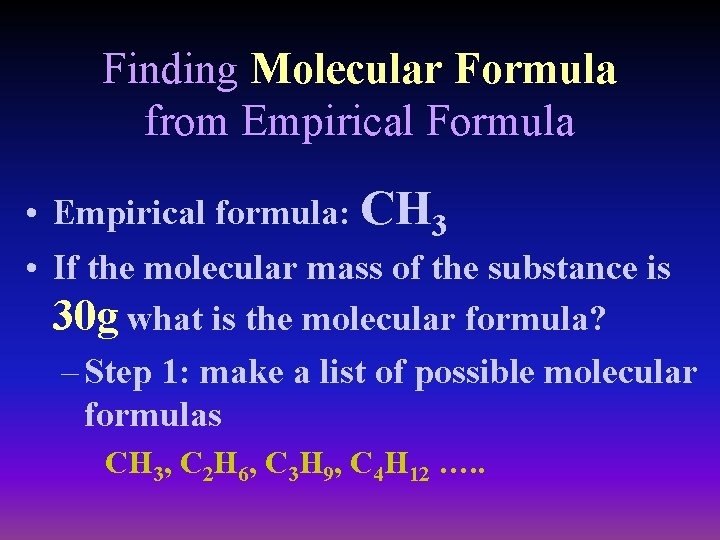Finding Molecular Formula from Empirical Formula • Empirical formula: CH 3 • If the molecular mass of the substance is 30 g what is the molecular formula? – Step 1: make a list of possible molecular formulas CH 3, C 2 H 6, C 3 H 9, C 4 H 12 …. .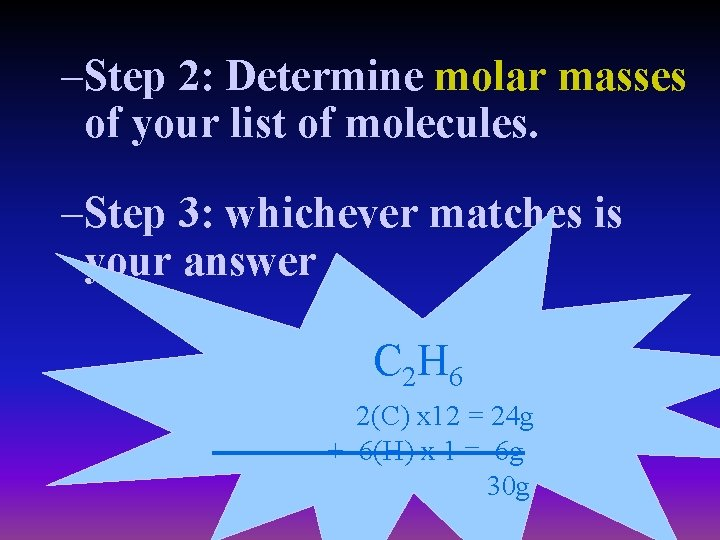–Step 2: Determine molar masses of your list of molecules. –Step 3: whichever matches is your answer C 2 H 6 2(C) x 12 = 24 g + 6(H) x 1 = 6 g 30 g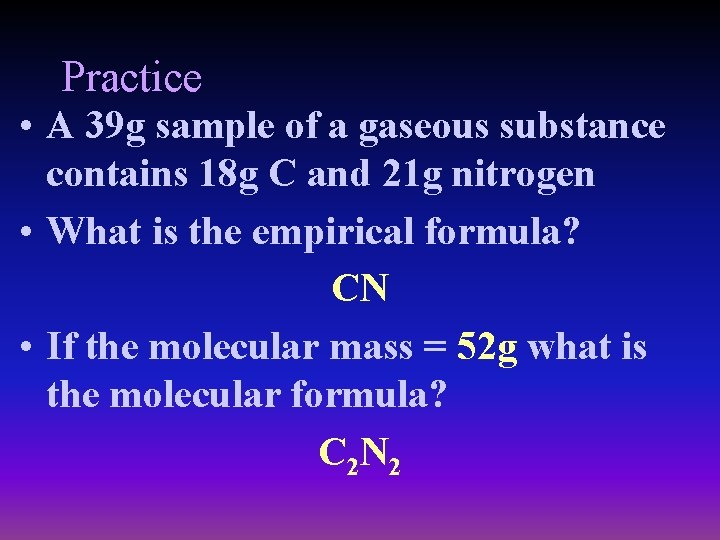Practice • A 39 g sample of a gaseous substance contains 18 g C and 21 g nitrogen • What is the empirical formula? CN • If the molecular mass = 52 g what is the molecular formula? C 2 N 2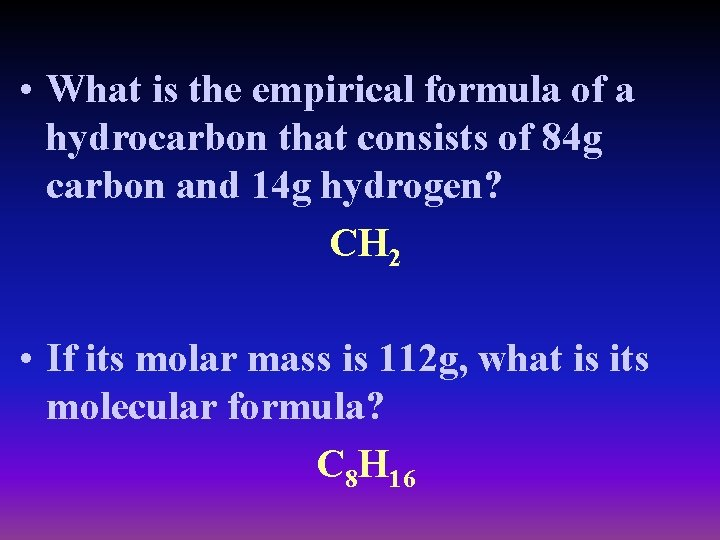• What is the empirical formula of a hydrocarbon that consists of 84 g carbon and 14 g hydrogen? CH 2 • If its molar mass is 112 g, what is its molecular formula? C 8 H 16• A compound known as a hydrate has the formula: K 2 Cr. O 4∙ 6 H 2 O. What percentage of this compound is water? 36%The END